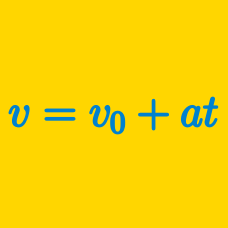Classical Mechanics

# Position Vector for Constant Velocity

Consider a point moving along a straight line. The position of the point is given by $p(t),$ where $t$ denotes elapsed time. If the point moves at a constant velocity of $2$ in the positive direction, and $p(0)=-1,$ what is the value of $p(7)?$

A ball is moving on a frictionless floor. If the initial velocity of the ball is $4.0\text{ m/s},$ what is the distance covered by the ball during $7.0$ seconds?

A point is moving along the $x$-axis. The initial position of the point is $7 \text{ cm},$ and the point is moving at a constant velocity of $-2\text{ cm s}^{-1}.$ What would be the position (in cm) of the point after $2$ seconds has passed?

Jake is on his bike heading home on a straight road. He is riding with a constant velocity of $5 \text{ m/s}.$ If he was initially $21 \text{ km}$ away from his home, and $40$ minutes has passed since then, how far is he from his home?

Earthquakes are motions of the earth's crust. Essentially, they are big sound waves that travel through the earth. There are two types of waves. The first type is called a longitudinal or P wave, where the earth compresses in the same direction as the wave travels. The second type is called a transverse, or S wave, where the compression in the earth is perpendicular to the direction of the wave. P waves travel roughly twice as fast as S waves.

A seismograph is a device for measuring these waves. By measuring the time between the arrival of each wave, we can calculate the distance to an earthquake. A seismograph measures a P wave and then an S wave 30 seconds later. If the P waves travel at 6000 m/s, how far away was the earthquake in kilometers?

Details and assumptions

• Assume the waves travel in straight lines.
×